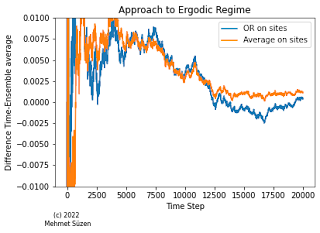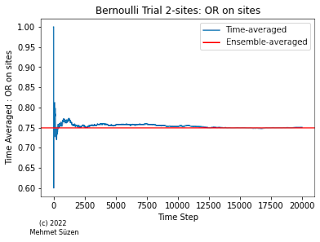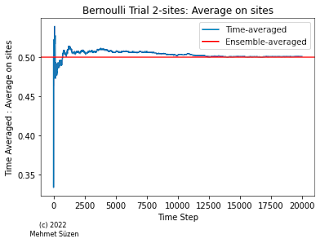## Wednesday, 11 May 2022

### A misconception in ergodicity: Identify ergodic regime not ergodic process

PreambleFigure 1: Two observable's approach to ergodicity for Bernoulli Trials.
Ergodicity appears in many fields, in physics, chemistry and natural sciences but in economics to machine learning as well. Recall that, ergodicity in physics and mathematical definition diverges significantly due to Birkhoff's statistical definition against Boltzmann's physical approach. Here we will follow Birkhoff's definition of ergodicity which is a statistical one. The basic notion of ergodicity is confusing even among experienced  academic circles. The primary misconception is that ergodicity is attributed to a process, a given process being ergodic. We address this by pointing out that ergodicity appears as a regime or a window so to speak for a given process's time-evolution and it can't be attributed to an entire generating process.

No such thing as ergodic process but ergodic regime given observable

A process being ergodic is not entirely true identification. Ergodicity is a regime over a given time window for a given observable derived from the process. This is the basis of ensemble theory from statistical physics.  Most of the processes generates initially a non-ergodic regime given an observable.  In order to identify an ergodic regime,  we need to define for a discrete setting :

1. the ensemble (sample space) : In discrete dynamics we also have an alphabet that ensemble is composed of.
2. an observable defined over the sample space.
3. a process (usually dynamics on the sample space evolving over-time).
4. a measure and threshold to discriminate ergodic to non-ergodic regimes.
Interesting thing is that different observables on the  same ensemble and the process may generate different ergodic regimes.

What are the processes and regime mathematically?

A process is essentially a dynamical system mathematically.  this includes stochastic models and as well as deterministic systems sensitive to initial conditions. Prominently these both combined in Statistical Physics. A regime mathematically implies a range of parameters or a time-window that a system behaves very differently.

Identification of ergodic regimeFigure 2: Evolution of time-averaged OR observable.
The main objective of finding out if dynamics produced by the process on our observable enters or in an ergodic regime is to measure if ensemble-averaged observable is equivalent to time-averaged observable value. Here equivalence is a difficult concept to address quantitatively. The simplest measure would be to check if $\Omega = \langle A \rangle_{ensemble} - \langle A \rangle_{time}$ is close to zero, i.e., vanishing. $\Omega$ being the ergodicity measure and $A$ is the observable with different averaging procedure. This is the definition we will use here. However, beware that in the physics literature there are more advanced measures to detect ergodicity, such as considering diffusion-like behaviour, meaning that the transition from non-ergodic to ergodic regime is not abrupt but have a diffusing approach to ergodicity.Figure 3: Evolution of time-averaged mean.
In some other academic fields approach to ergodic regime has different names not strictly but closely related, such as in chemical physics or molecular dynamics, equilibration time, relaxation time, equilibrium, steady-state for a given observable, in statistics Monte Carlo simulations, it is usually called burn out period. Not always, but in ergodic regime, observable is stationary and time-independent. In Physics, this is much easier to distinguish because time-dependence, equilibrium and stationarity are tied to energy transfer to the system.

Ergodic regime not ergodic process : An example of Bernoulli Trials

Apart from real physical processes such as Ising Model, a basic process we can use to understand how ergodic regime could be detected using Bernoulli Trials.

Here for a Bernoulli trials/process, we will use random number generators for a binary outcome, i.e., RNG Marsenne-Twister  to generate time evolution of an observables on two sites:  Let's say we have two sites  $x, y \in \{1, 0\}$.  The ensemble of this two site system $xy$ is simply the sample space of all possible outcomes $S=\{10, 11, 01, 00\}$. Time evolution of such two site system is formulated here as choosing $\{0,1\}$  for a given site at a given time, see Appendix Python notebook.

Now, the most important part of checking ergodic regime is that we need to define an observable over two side trials. We denote two observable as $O_{1}$, which is an OR operation between sites, and $O_{2}$ is averaged over two sites. Since our sample space is small, we can compute the ensemble average observables analytically:

• $O_{1} = (x+y)/2$  then  $10, 11, 01, 00 ; (1/2 + 2/2 + 1/2 + 0 ) /4 = 0.5$
• $O_{2} = x OR y$ then   $10, 11, 01, 00 ; ( 1 + 1 + 1 + 0 )/4 = 0.75$

We can compute the time-averaged observables over time via simulations, but their formulation are know as follows:

•  Time average for $O_{1}$ at time $t$  (current step)  is   $\frac{1}{t} \sum_{i=0}^{t} (x_{i}+y_{i})/2.0$
• Time average for $O_{2}$ at time $t$  (current step)  is   $\frac{1}{t} \sum_{i=0}^{t} (x_{i} OR y_{i})$.

One of the possible trajectories are shown in Figure 2 and 3. For approach to ergodicity measure, we shown this at Figure 1. Even though, we should run multiple trajectories to have error estimates, we can clearly see that ergodicity regime starts after 10K steps, at least. Moreover, different observables have different decay rates to ergodic regime.  From preliminary simulation, it appears to be OR observable converges slower, though this is a single trajectory.

Conclusion

We have shown that manifestation of the ergodic regime depends on the time-evolution of the observable given a measure of ergodicity, i.e., a condition how ergodicity is detected. This exposition should clarify that a generating process does not get an attribute of "ergodic process" rather we talk about "ergodic regime" depending on observable and the process over temporal evolution. Interestingly, from Physics point of view, it is perfectly possible that an observable attains ergodic regime and then falls back to non-ergodic regime.